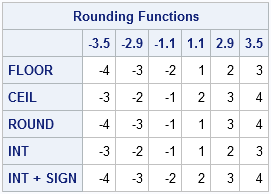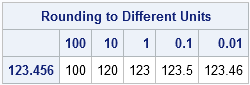SAS has several ways to round a number to an integer. You can round a number up, round it down, or round it to the nearest integer. If your data contain both positive and negative values, you can also round numbers toward zero, or away from zero.

The functions that perform rounding are the same in the DATA step as in the SAS/IML language:

The following statements evaluate each function on a vector of numbers so that you can observe the different rounding behaviors:

```proc iml; x={-3.5 -2.9 -1.1 1.1 2.9 3.5}; f = floor(x); /* towards -infinity */ c = ceil(x); /* towards +infinity */ r = round(x); /* towards nearest integer */ t = int(x); /* towards zero */ s = int(x) + sign(x); /* away from zero */   m = f//c//r//t//s; /* pack into matrix */ cNames = putn(x,"BEST4."); rNames = {FLOOR CEIL ROUND INT "INT + SIGN"}; print m[c=cNames r=rNames label="Rounding Functions"];```### Rounding to units that are not integers

Some SAS users do not realize that the ROUND function enables you to round a number to a certain number of decimal places. The ROUND function takes an optional second argument, which is the rounding unit. The following statements round a number to the nearest hundred, the nearest ten, the nearest unit (the default), the nearest tenth, and the nearest hundredth:

```x = 123.456; units = {100 10 1 0.1 0.01}; y = round(x, units); cNames = putn(units,"BEST4."); print y[c=cNames r="123.456" label="Rounding to Different Units"];```Although it is not usually done, you can also round a number to any unit you want. For example, round(x,1/3) rounds x to the nearest third.

ShareDistinguished Researcher in Computational Statistics

Rick Wicklin, PhD, is a distinguished researcher in computational statistics at SAS and is a principal developer of SAS/IML software. His areas of expertise include computational statistics, simulation, statistical graphics, and modern methods in statistical data analysis. Rick is author of the books Statistical Programming with SAS/IML Software and Simulating Data with SAS.

1.Michael Richardson on

One caveat to your "round away from zero" function: notice that integer values would be rounded to the next integer from 0 ('3' is rounded to '4'). I doubt that would be the user's intention. Perhaps
s = ceil(abs(x)) # sign(x);
is a better choice.

2.Is there a way to always round down to different units? For example, I might want 199 to round down to 100.

•Yes. The ROUND function has a second optional argument that specifies the rounding unit. Therefore ROUND(x, 100) rounds x to the nearest 100. To round DOWN, you'll need to subtract off half of the rounding unit. Thus ROUND(x-50, 100) rounds x down to the nearest multiple of 100.

•Can you explain algorithm of round function?

•The documentation (which I link to in the article) contain a complete description. Briefly, ROUND(x) returns the closest integer to x. ROUND(x, 0.1) returns the nearest "tenth" to x.

3.Hi Rick, how could we ceil/floor a number to decimal points? For example, ceil 1.88 to 1.9 rather than 2.

•To round, you can use round(1.88, 0.1). To floor or ceil), you can first multiply by the rounding factor, apply floor/ceil, and then divide by the rounding factor. In your case, the answer is ceil(10*1.88) / 10. If you want two decimal places: ceil(100*x)/100. For k decimal places: ceil(10**k * x)/ 10**k.

4."SAS does not have a built-in function that rounds away from zero, but you can combine the SIGN function with another function to round away from zero". That "another function" could be truncn() - a user-defined function that truncates decimal numbers. It is described in Truncating decimal numbers in SAS without rounding. One can add sign() function (that returns -1 for negative, +1 for positive, and 0 for zero) to the truncated number to achieve rounding away from zero:
truncn(x) + sign(x).

5.Hi Rick! I want to round all of the values of a variable up to the nearest 100. For example 135 to 200. Is there a way to do this using this function?

•Sure. If you want to round to the nearest quantity, Q, use Q*ceil(x/Q). The following illustrates Q=100:

```data A; input x @@; R = 100 * ceil( x / 100 ); datalines; 2 97 100 101 135 199 200 201 ; proc print; run;```
•Worked perfectly, thank you so much!!

•Hi Rick,

If I want round down 195 to 100, 215 to 200,285 to 200 Which function I can use?

•To round down to the nearest 100, you need to
1. Divide your number by 100.
2. Use the FLOOR function to round down the ratio to the nearest integer
3. Multiply the result by 100 to restore the scale of the original number.
If x is your number, the SAS statement looks like this
y = 100 * floor(x/100); /* round down to nearest 100 */

6.Patrick O'Leary on

Hi Rick! I would like to round up to the nearest 0.003. Example : 0.051 would become 0.053.
Than you!

•The expression y = round(x, 0.003) rounds x to the nearest multiple of 0.003. However, the nearest multiple of 0.051 is itself because 0.051 = (17)(0.003). The next multiple is 0.054, so nothing will ever round to 0.053.

7. Pingback: Round to even - The DO Loop

8.Hi Rick,
In a single column, how can I round all negative numbers to zero but keep all positive numbers as they are?

•Since this sounds like a homework/test problem, I'll just give a hint. The solution does not use rounding functions. You are looking for a transformation that takes negative numbers to zero and positive numbers to themselves. If you think about the graph of such a function, that will show you how to implement it.

9.Hi Rick,
Is there a way to round down like this , 2.325 to 2.32 instead of 2.33?

•Are all 0.5s rounded down, or just some? If you are talking about the Round-To-Even rule, then use the ROUNDE function.

•All 5's need to be rounded down. All 0.5's I mean.

•Yes. There are actually two methods, depending on how you want to handle negative numbers. You probably want the "round half down" method, which rounds all 0.5 towards negative infinity:

```data RoundHalfDown; input x @@; RndDown = floor( ceil(2*x)/2 ); /* round half towards negative infinity */ datalines; -0.51 -0.5 -0.49 0 0.49 0.5 0.51 2 2.5 2.51 ;   proc print; run;```
10.hi , how do you round up to the nearest 10 in a sas dataset. For example , round up 132 to 140.

•See my response to Erin on January 10, 2018.

11.Thanks Rick, it worked :)

12.Can I write round(var, 0.005) to 0.005 * ceil(var/0.005) ?

•No. For example, var=1.001 does not work.

13.Hi Rick,

I have data of some 900 odd obs. The total of that comes out to be 100k. Some of the values are upto 6 decimal places. The client wants the values to be up to 2 decimal places. but when I use a round function(round(var, .01)) or int function or ceil or floor function, the total is either less or more than 100k. is there any other function or multiple conditions i can use so that the total always comes out to be 100k. Thank you in advance.

•When you round, you are changing the data, so that changes the sum. Instead, apply a SAS w.d format such as 10.2. That changes the way that the values are displayed but does not change the sums.

•Hi Rick, thank you for your response.

I am already using format 10.2 so its displayed up to 2 decimal places. When I export the report to excel, in the cell the value is displayed upto 2 decimal places but in the function bar in ms excel it shows the original value which is up to 6 decimal places. I tried truncating the values by converting it into character & using substr function to show the value upto 2 decimal places but the total is always more or less than 100k. i want the value in the function bar as well to be upto 2 decimal places & the total matching to 100k.